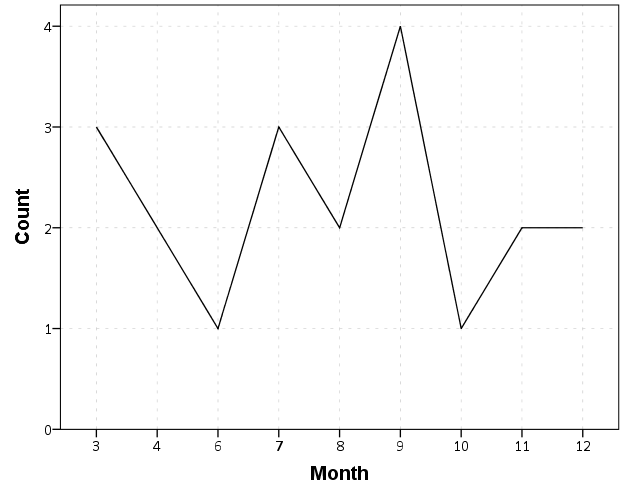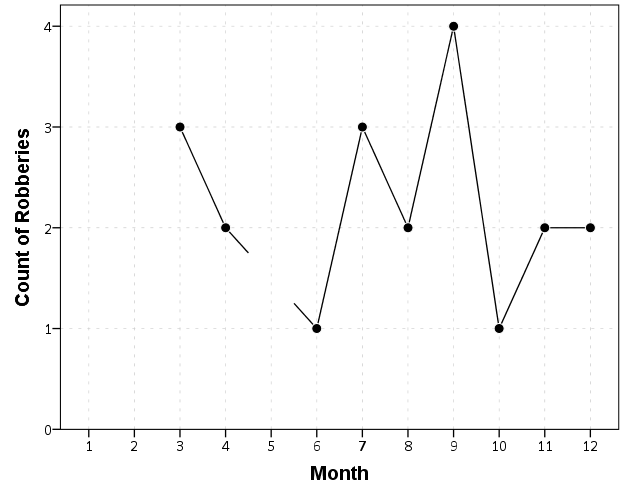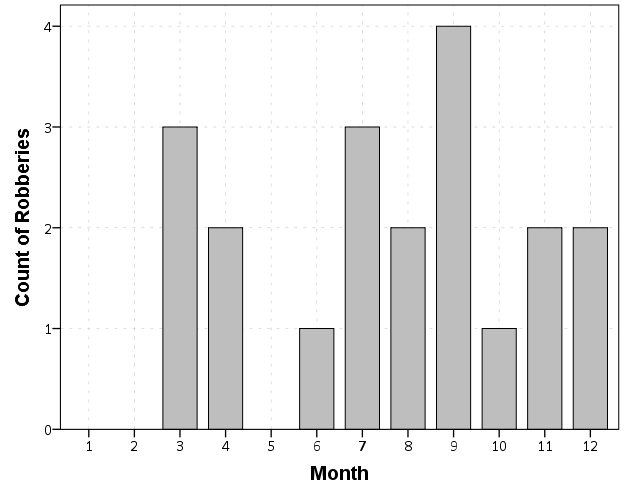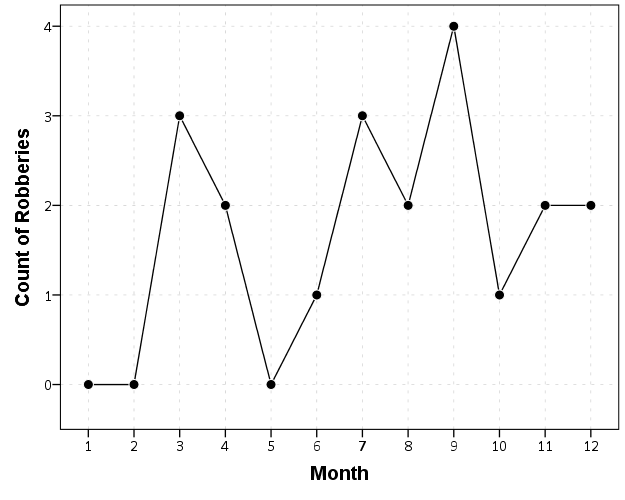One common task I undertake in is to make time series graphs of crime counts, often over months or shorter time periods. Here is some example data to illustrate, a set of 20 crimes with a particular date in 2013.

``````*Make some fake data.
SET SEED 10.
INPUT PROGRAM.
LOOP #i = 1 TO 20.
COMPUTE #R = RV.UNIFORM(0,364).
COMPUTE DateRob = DATESUM(DATE.MDY(1,1,2013),#R,"DAYS").
END CASE.
END LOOP.
END FILE.
END INPUT PROGRAM.
EXECUTE.``````

SPSS has some convenient functions to aggregate right within `GGRAPH`, so if I want a chart of the number of crimes per month I can create my own `Month` variable and aggregate. The pasted `GGRAPH` code is generated directly though the Chart Builder GUI.

``````COMPUTE Month = XDATE.MONTH(DateRob).
FORMATS Month (F2.0).

*Default Line chart.
GGRAPH
/GRAPHDATASET NAME="graphdataset" VARIABLES=Month COUNT()[name="COUNT"] MISSING=LISTWISE
REPORTMISSING=NO
/GRAPHSPEC SOURCE=INLINE.
BEGIN GPL
SOURCE: s=userSource(id("graphdataset"))
DATA: Month=col(source(s), name("Month"), unit.category())
DATA: COUNT=col(source(s), name("COUNT"))
GUIDE: axis(dim(1), label("Month"))
GUIDE: axis(dim(2), label("Count"))
SCALE: linear(dim(2), include(0))
ELEMENT: line(position(Month*COUNT), missing.wings())
END GPL.``````So at first glance that looks alright, but notice that the month’s do not start until 3. Also if you look close you will see a 5 is missing. What happens is that to conduct the aggregation in `GGRAPH`, SPSS needs to treat `Month` as a categorical variable – not a continuous one. SPSS only knows of the existence of categories contained in the data. (A similar thing happens in `GROUP BY` statements in SQL.) So SPSS just omits those categories.

We can manually specify all of the month categories in the axis. To reinforce where the measurements come from I also plot the points on top of the line.

``````*Line chart with points easier to see.
GGRAPH
/GRAPHDATASET NAME="graphdataset" VARIABLES=Month COUNT()[name="COUNT"] MISSING=LISTWISE
REPORTMISSING=NO
/GRAPHSPEC SOURCE=INLINE.
BEGIN GPL
SOURCE: s=userSource(id("graphdataset"))
DATA: Month=col(source(s), name("Month"), unit.category())
DATA: COUNT=col(source(s), name("COUNT"))
GUIDE: axis(dim(1), label("Month"))
GUIDE: axis(dim(2), label("Count of Robberies"))
SCALE: cat(dim(1), include("1","2","3","4","5","6","7","8","9","10","11","12"))
SCALE: linear(dim(2), include(0))
ELEMENT: line(position(Month*COUNT), missing.wings())
ELEMENT: point(position(Month*COUNT), color.interior(color.black),
color.exterior(color.white), size(size."10"))
END GPL.``````So you can see that `include` statement with all of the month numbers. You can also see what that mysterious `missing.wings()` function actually does in this example. It is misleading though, as 5 isn’t missing, it is simply zero.

A simple workaround for this example is to just use a bar chart. A zero bar is not misleading.

``````GGRAPH
/GRAPHDATASET NAME="graphdataset" VARIABLES=Month COUNT()[name="COUNT"] MISSING=LISTWISE
REPORTMISSING=NO
/GRAPHSPEC SOURCE=INLINE.
BEGIN GPL
SOURCE: s=userSource(id("graphdataset"))
DATA: Month=col(source(s), name("Month"), unit.category())
DATA: COUNT=col(source(s), name("COUNT"))
GUIDE: axis(dim(1), label("Month"))
GUIDE: axis(dim(2), label("Count of Robberies"))
SCALE: cat(dim(1), include("1","2","3","4","5","6","7","8","9","10","11","12"))
SCALE: linear(dim(2), include(0))
ELEMENT: interval(position(Month*COUNT))
END GPL.``````I often prefer line charts for several reasons though, often to superimpose multiple lines (e.g. I may want to put the lines for counts of crimes in 2012 and 2011 as well). Line charts are clearly superior to clustered bar charts in that situation. Also I prefer to be able to keep time is a numerical variable in the charts, and one can’t do that with aggregation in `GGRAPH`.

So I do the aggregation myself.

``````*Make a new dataset.
DATASET DECLARE AggRob.
AGGREGATE OUTFILE='AggRob'
/BREAK = Month
/CountRob = N.
DATASET ACTIVATE AggRob.``````

But we have the same problem here, in that months with zero counts are not in the data. To fill in the zeroes, I typically make a new dataset of the date ranges using `INPUT PROGRAM` and loops, same as I did to make the fake data at the beginning of the post.

``````*Make a new dataset to expand to missing months.
INPUT PROGRAM.
LOOP #i = 1 TO 12.
COMPUTE Month = #i.
END CASE.
END LOOP.
END FILE.
END INPUT PROGRAM.
DATASET NAME TempMonExpan.``````

Now we can simply merge this expanded dataset back into `AggRob`, and the recode the system missing values to zero.

``````*File merge back into AggRob.
DATASET ACTIVATE AggRob.
MATCH FILES FILE = *
/FILE = 'TempMonExpan'
/BY Month.
DATASET CLOSE TempMonExpan.
RECODE CountRob (SYSMIS = 0).``````

Now we can make our nice line chart with the zeros in place.

``````GGRAPH
/GRAPHDATASET NAME="graphdataset" VARIABLES=Month CountRob
/GRAPHSPEC SOURCE=INLINE.
BEGIN GPL
SOURCE: s=userSource(id("graphdataset"))
DATA: Month=col(source(s), name("Month"))
DATA: CountRob=col(source(s), name("CountRob"))
GUIDE: axis(dim(1), label("Month"), delta(1), start(1))
GUIDE: axis(dim(2), label("Count of Robberies"), start(0))
SCALE: linear(dim(1), min(1), max(12))
SCALE: linear(dim(2), min(-0.5))
ELEMENT: line(position(Month*CountRob))
ELEMENT: point(position(Month*CountRob), color.interior(color.black),
color.exterior(color.white), size(size."10"))
END GPL.``````To ease making these separate time series datasets I have made a set of macros, one named `!TimeExpand` and the other named `!DateExpand`. Both take a begin and end date and then make an expanded dataset of times. The difference between the two is that `!TimeExpand` takes a user specified step size, and `!DateExpand` takes a string of the types used in SPSS date time calculations. The situation in which I like to use `!TimeExpand` is when I do weekly aggregations from a specified start time (e.g. the weeks don’t start over at the beginning of the year). It also works for irregular times though, say if you wanted 15 minute bins. `!DateExpand` can take years, quarters, months, weeks, days, hours, minutes, and seconds. The end dates can also be system variables like `\$TIME` as well. The macro can be found here, and it contains several examples within. Update: I have added a few macros that do the same thing for panel data. It just needs to take one numeric variable as the panel id, otherwise the argument for the macros are the same.